# C++ Program To Implement Selection Sort

Do you know what is “Selection Sort in C++“? Have you ever thought what are the steps to develop the Selection Sort in C++? Let us Gain some knowledge about the Selection sort in C++.

You should be aware that different types of sorting algorithms are present in the Data Structures. But have
you ever come across the Selection Sort Algorithm concept? Do you know what is the process for
implementing the Selection Sort Technique in C++ programming language?

In this article, we are going to discuss the Selection Sort Algorithm from scratch to build a good knowledge
of the topic. So, let us start our discussion.

## What Is Selection Sort Algorithm? Get To Know

Sorting algorithms are the most important algorithms. There are many algorithms. They all have a different strategies to sort the giving sequence. Like there is the Quick sort algorithm, Bubble sort algorithm, Heap sort algorithm, etc. Like those sorting algorithms, there is another famous present. This is known as the Selection sort algorithm.

In this algorithm, a special element is selected. Sometimes the starting element or the last element is considered the required element. Now, based on the comparison of the elements, we have to get the minimum element present in the sequence.

### What Is The Pseudo-Code Of Selection Sort Algorithm?

Now, before moving to the step-by-step implementation process of the Selection Sorting Technique, it is
time to know the Pseudo-Code of the algorithm. Let us find out the Pseudo-Code below:

```				```
func. selectionSort (a, n)
execute loop (n - 1) times
Take the first element as the minimum
execute loop for each of the unsorted elements
if element < minimum
make element as new-minimum
swap position of minimum with the first position
end func. selectionSort
```
```

### How Does Selection Sort Work?

After getting knowledge about the Selection sort algorithm, it is time to know the working procedure of the
Selection Sort in C++. So, we need to have an array with integer elements. The array will be in a completely
unsorted format. Using the Selection Sort algorithm, we can change it to the sorted one.

Let us take one example of selection sort in C with example. Suppose, in an array, there are three elements.
They are 2,3,1. As we can see they are in the unsorted format. Now, if we want to make them in the sorted
form, we have to use the theory of Selection Sort.

##### Step 1:

At first, we will consider the beginning element as the minimum one. So, as per the theory, element
2 is the minimum one.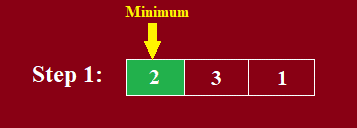##### Step 2:

Now, we have to find out the real minimum number from this array. So, we will run a for loop to
check each & every element. Now, while checking with element 3, element 2 is still the minimum one.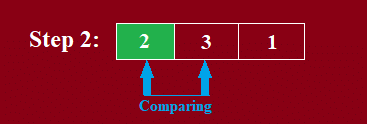##### Step 3:

So, the minimum tag will remain upon element 2.##### Step 4:

Now, again we have to check element 2 with element 1. Now, element 1 is lower than element 2. So,
the minimum tag will move to element 1.##### Step 5:

As the minimum tag is on element 1, we have to make some changes in the array. The element
which has the minimum tag will always be placed at the beginning of the array. So, element 2 & element 1
will change their place. Now, the minimum tag will move to element 3.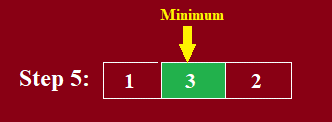##### Step 6:

Now, there will be a comparison between Element 3 and Element 2. There we have to find out
which element is minimum.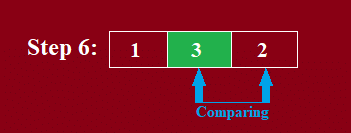##### Step 7:

As element 2 is the minimum in this comparison, the swap of passion will again happen. Element 2
will take the place of Element 3. In this way, we have found the sorted array using Selection Sort in C++.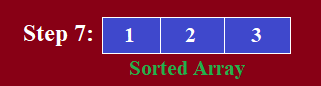### What Is The Implementation Of Selection Sort In C++?

Now, after knowing about the steps of the Selection sort, it is time to move forward. Now, we will try to
implement the Selection sort in C++. The steps of the implementation process of the selection sort algorithm
are the following:
1. At first, in the main function, the array of the elements will be declared. The elements are present in
an unsorted manner.
2. Now, the first element will be used as the minimum element for moving ahead.
3. Now, another user-defined function will be called to print the array. As it is an unsorted array, the
same pattern will be printed.
4. Now, the user-defined function for the selection sort algorithm will be used. Here, a for loop will be
used that will check the elements concerning the minimum elements.
5. Based on the result, the elements will be swapped & minimum elements will be changed.
6. Now, again the print function will be called. Hence, the new sorted array will be printed.

##### Code To Demonstrate The Implementation Process Of Selection Sort In CPP:
```				```
#include<bits/stdc++.h> using namespace std;
void printzap(int cod[], int n){// PrintingFunction
for(int i=0;i<n;i++)
cout<<cod[i]<<" ";
cout<<endl;}
void Sort(int cod[], int n){// Sorting Function
int i,j,min;
for(i=0;i<n;i++){// Finding The Minimum Element & Swapping
min = i;
for(j=i+1;j<n;j++)
if (cod[j] < cod[min])
min = j;
swap(cod[i],cod[min]);}}// Swapping The Element
int main(int argv, char* argc[]){ int zap[] = {2,3,10,4,1};// Declaring The Array Void
int i,j,n,temp; n = sizeof(zap)/sizeof(int);// Finding The Size
cout<<"Unsorted Array: "; printzap(zap,n);// Printing The Unsorted Array
Sort(zap,n);// Sorting Function
cout<<"Sorted Array: "; printzap(zap,n);// Printing The Sorted Array
return0;}
```
```

Let us find the output of the above code. This will help to understand the Selection Sort in C++.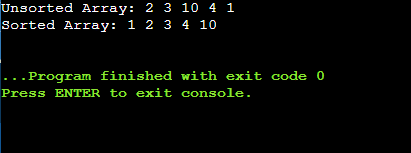From the above output, we can see that the unsorted array is printed first. After performing all the
operations, the array becomes the sorted one & it is printed. Hence, the Selection Sort Algorithm is working
fine.

### What Is The Time Complexity Of Selection Sort Algorithm?

The time complexity of the Selection Sort is O(n2). There are mainly three complexity parameters present.
They are the Best-Case, Average-Case, and Worst-Case time complexity. In Selection Sort all there three
parameters have the same time complexity. This is the O(n2).

## Conclusion:

As we see Selection sort in C++ is a very important topic.
We have to remember how selection sort work & selection sort in C with example in a good manner.
To get knowledge about the Selection Sort Cpp, we need to first clear the basics of Data Structures and
Algorithms.